# Recursion n Recursive definitions n Recursive methods n

• Slides: 18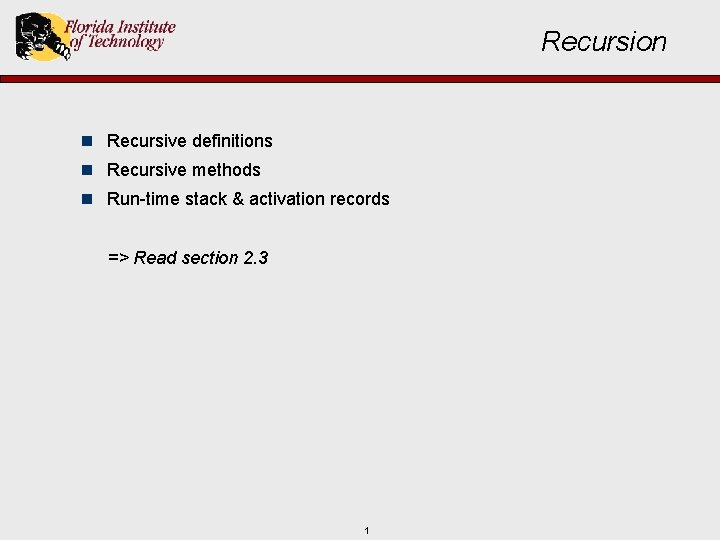Recursion n Recursive definitions n Recursive methods n Run-time stack & activation records => Read section 2. 3 1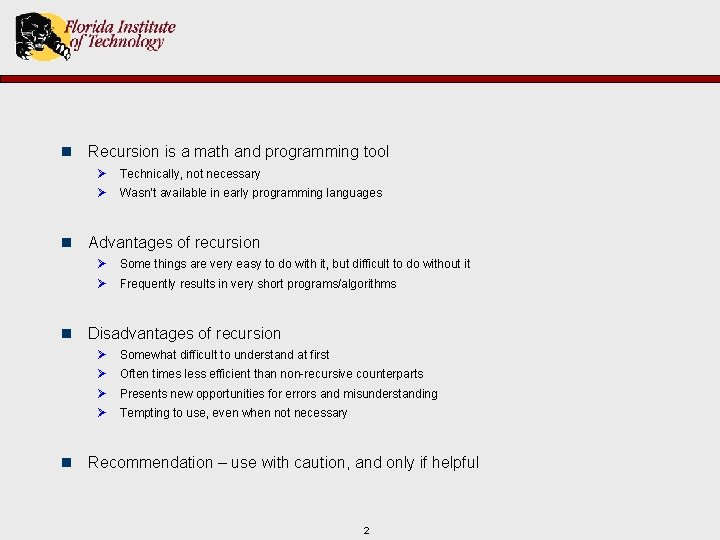n Recursion is a math and programming tool Ø Technically, not necessary Ø Wasn’t available in early programming languages n Advantages of recursion Ø Some things are very easy to do with it, but difficult to do without it Ø Frequently results in very short programs/algorithms n Disadvantages of recursion Ø Ø Somewhat difficult to understand at first Often times less efficient than non-recursive counterparts Presents new opportunities for errors and misunderstanding Tempting to use, even when not necessary n Recommendation – use with caution, and only if helpful 2Recursive Definitions n Factorial – Non-recursive Definition: N! = N * (N-1) * (N-2) * … * 2 * 1 *Note that a corresponding Java program is easy to write public static int fact(int n) : 3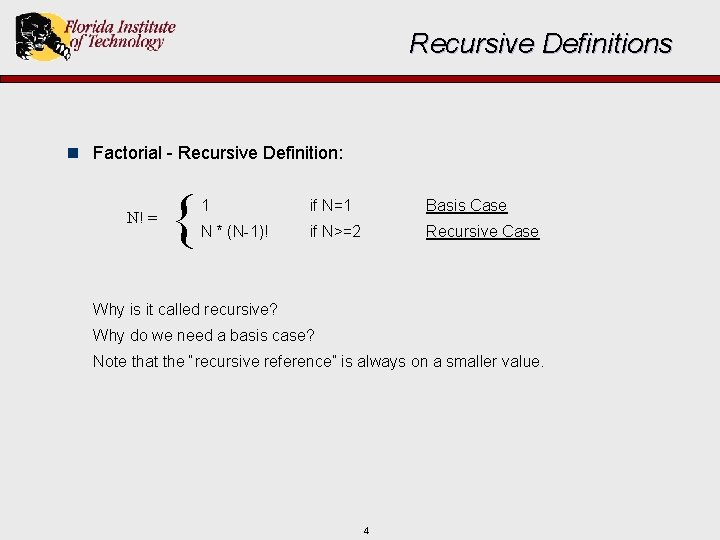Recursive Definitions n Factorial - Recursive Definition: N! = { 1 if N=1 Basis Case N * (N-1)! if N>=2 Recursive Case Why is it called recursive? Why do we need a basis case? Note that the “recursive reference” is always on a smaller value. 4Recursive Definitions n Fibonacci - Non-Recursive Definition: 0 1 1 2 3 5 8 13 21 34 … *Note that a corresponding Java program is easy to write…or is it? public static int fib(int n) : 5Recursive Definitions n Fibonacci - Recursive Definition: fib(N) = { 0 if N=1 Basis Case 1 if N=2 Basis Case fib(N-1) + fib(N-2) if N>=3 Recursive Case Note there are two basis cases and two recursive references. 6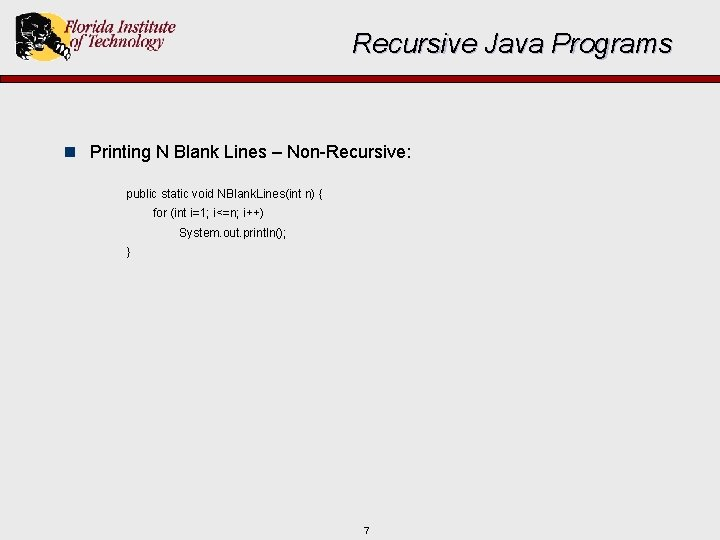Recursive Java Programs n Printing N Blank Lines – Non-Recursive: public static void NBlank. Lines(int n) { for (int i=1; i<=n; i++) System. out. println(); } 7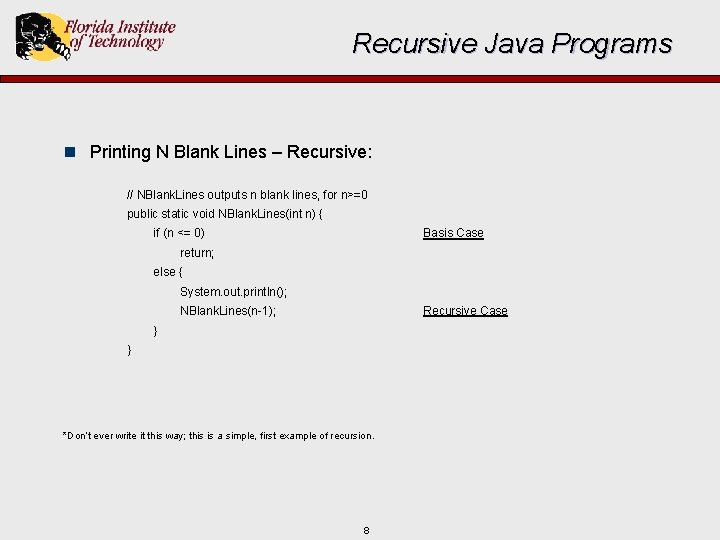Recursive Java Programs n Printing N Blank Lines – Recursive: // NBlank. Lines outputs n blank lines, for n>=0 public static void NBlank. Lines(int n) { if (n <= 0) Basis Case return; else { System. out. println(); NBlank. Lines(n-1); Recursive Case } } *Don’t ever write it this way; this is a simple, first example of recursion. 8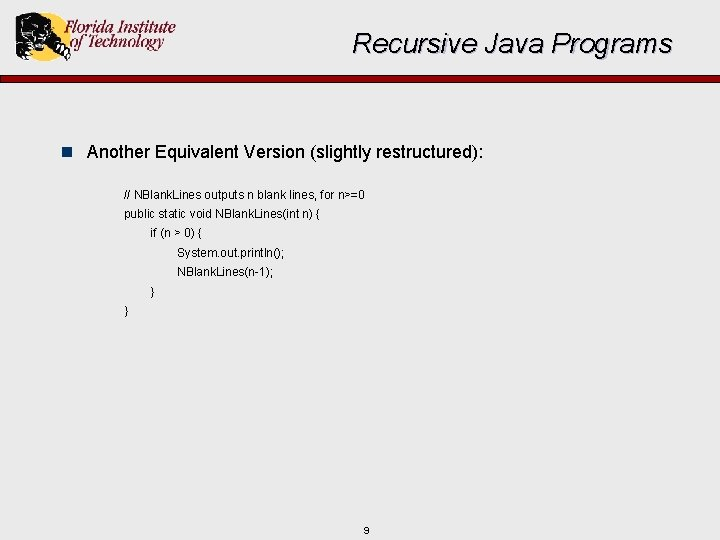Recursive Java Programs n Another Equivalent Version (slightly restructured): // NBlank. Lines outputs n blank lines, for n>=0 public static void NBlank. Lines(int n) { if (n > 0) { System. out. println(); NBlank. Lines(n-1); } } 9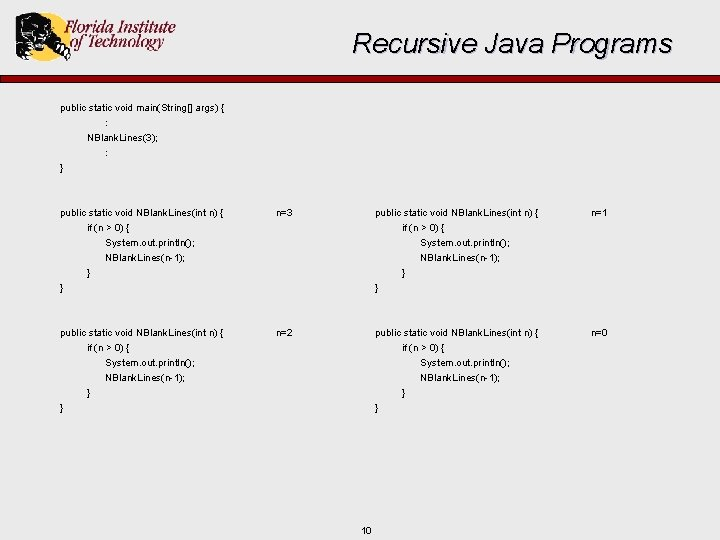Recursive Java Programs public static void main(String[] args) { : NBlank. Lines(3); : } public static void NBlank. Lines(int n) { if (n > 0) { System. out. println(); NBlank. Lines(n-1); } } n=3 public static void NBlank. Lines(int n) { if (n > 0) { System. out. println(); NBlank. Lines(n-1); } } n=1 public static void NBlank. Lines(int n) { if (n > 0) { n=2 public static void NBlank. Lines(int n) { if (n > 0) { System. out. println(); NBlank. Lines(n-1); } } n=0 System. out. println(); NBlank. Lines(n-1); } } 10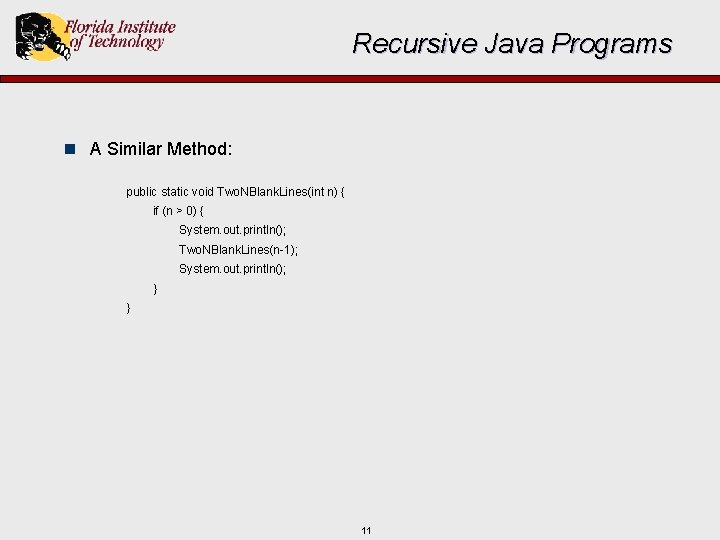Recursive Java Programs n A Similar Method: public static void Two. NBlank. Lines(int n) { if (n > 0) { System. out. println(); Two. NBlank. Lines(n-1); System. out. println(); } } 11Recursive Java Programs public static void main(String[] args) { : Two. NBlank. Lines(2); : } public static void Two. NBlank. Lines(int n) { if (n > 0) { System. out. println(); Two. NBlank. Lines(n-1); System. out. println(); } } n=2 public static void Two. NBlank. Lines(int n) { n=1 public static void Two. NBlank. Lines(int n) { if (n > 0) { System. out. println(); Two. NBlank. Lines(n-1); System. out. println(); } } 12 n=0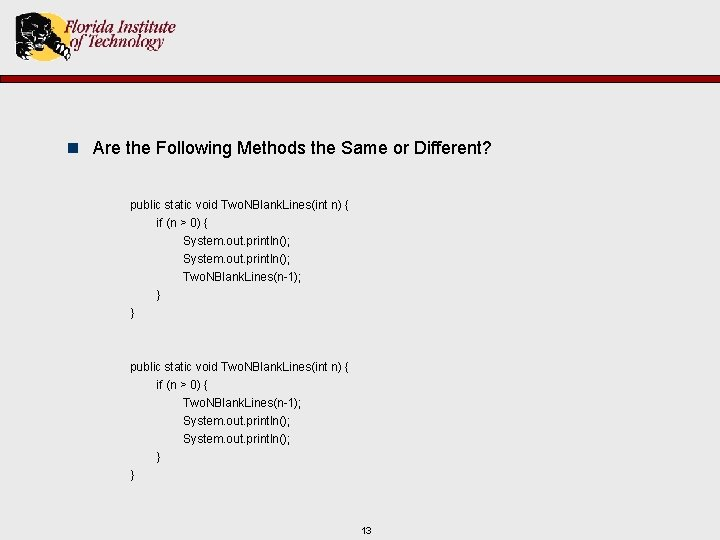n Are the Following Methods the Same or Different? public static void Two. NBlank. Lines(int n) { if (n > 0) { System. out. println(); Two. NBlank. Lines(n-1); } } public static void Two. NBlank. Lines(int n) { if (n > 0) { Two. NBlank. Lines(n-1); System. out. println(); } } 13n Recursive Factorial Definition: N! = { 1 N * (N-1)! if N=1 Basis Case if N>=2 Recursive Case n Recursive Factorial Program: public static int fact (int n) { if (n==1) return 1; else { int x; x = fact (n-1); return x*n; Basis Case Recursive Case } } 14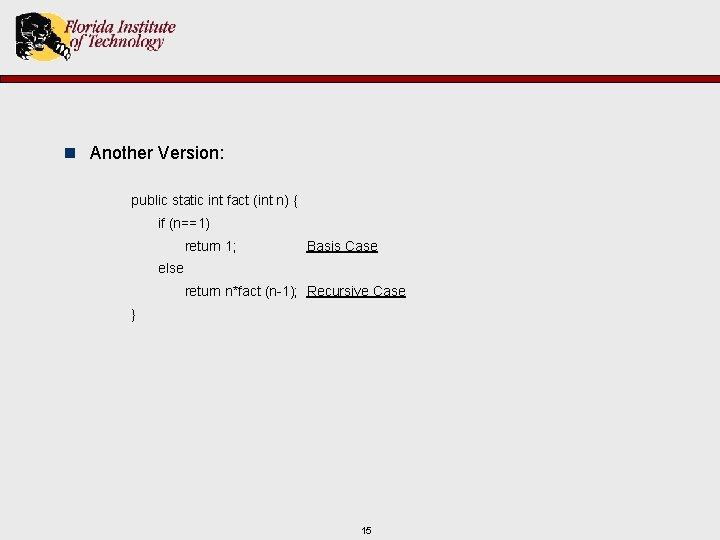n Another Version: public static int fact (int n) { if (n==1) return 1; Basis Case else return n*fact (n-1); Recursive Case } 15n Recursive Fibonacci Definition: fib(N) = { 0 if N=1 Basis Case 1 if N=2 Basis Case fib(N-1) + fib(N-2) if N>=3 Recursive Case n Recursive Fibonacci Program: public static int fib (int n) { if (n==1) return 0; else if (n==2) return 1; else { int x, y; x = fib (n-1); y = fib (n-2); return x+y; } } Basis Case Recursive Case 16n Another Version: public static int fib (int n) { if (n==1) return 0; else if (n==2) return 1; else return fib(n-1) + fib(n-2); } 17Recursion & the Run-time Stack n How does recursion related to stack frames and the run time stack? Ø Note that stack frames are sometimes called allocation records or activation records n Why might a recursive program be less efficient than nonrecursive counterpart? n Why is the recursive fibonnaci function especially inefficient? 18